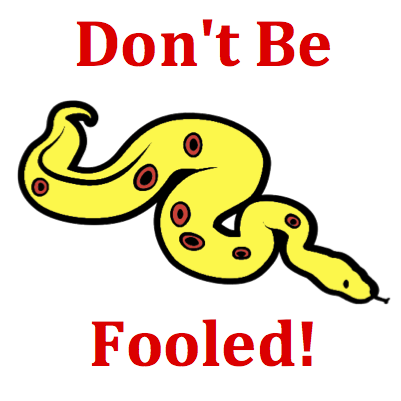# Kinematic Graphing - Mission KG6 Detailed HelpThe motion of an object is depicted by a velocity-time graph. Various time intervals during the motion are depicted by a letter. During which time interval(s) is the object moving with a negative acceleration? List all that apply ... .Velocity-Time Graphs: Velocity versus time graphs represent changes that occur in an object's velocity with respect to time. The slope of the line is the acceleration (change in velocity divided by the change in time) of the object. The area under the line (between the line on the graph and the time axis) is the displacement of the object.Many students confuse position-time graphs with velocity-time graphs. They know that an acceleration on a position-time graph as a curved line. So they instantly begin looking for the same line on this graph. But don't be fooled! This is a velocity-time graph and the slope is the acceleration. A line with changing slope (curved line) on a velocity-time graph is a line with a changing acceleration; but it is not the only way to represent an acceleration (see the Think About It section).The slope of the line on a velocity-time graph is the acceleration (see Know the Law section). As the slope goes, so goes the acceleration. A line with a slope (not horizontal) represents an object with an acceleration. And a line with a slope which is negative represents an object with a negative acceleration.